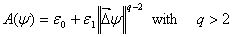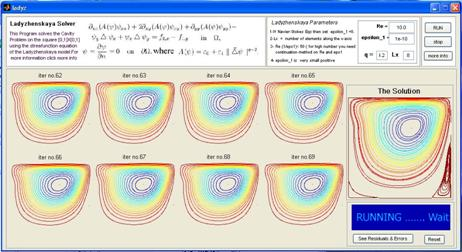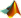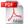﻿ FEMLADYNumerical Solution for the Ladyzhenskaya Equations (FEMLADY) Tables of Contents 1) WHAT IS FEMLADY 2) HOW TO USE FEMLADY 3) MATLAB & FORTRAN FILES 1) WHAT IS FEMLADY: FEMLADY is a FORTRAN77 program, using double precision arithmetic, which solves the Ladyzhenskaya equations in the pure streamfunction version describing the flow of an incompressible fluid.whereand*  If we setthe above equaton reduces to the streamfunction equation of the Navier-Stokes equations. *  This program uses the Finite Element Method with the Bogner-Fox-Schmit rectangles to solve the driven cavity problem in the 2-dimensional square [0,1]X[0,1] with no slip boundary conditions, i.e., u1=u2=0  in all boundaries except y=1, where u1=1. *  The program can solve the Navier-Stokes equations and the Smagorinsky model and the Biharmonic equation. * The program uses the GUI MATLAB as interface method to communicate friendly with the users. Also it uses all graphing utilities in MATLAB to visualize the solution of the problem. * Fig. 1  shows the first window of the programFig. 1 2) HOW TO USE FEMLADY: Copy the FEMLADY  folder in your hard disk and within MATLAB command go to FEMLADY folder  and just type ladyz the above window will show. Then enter the parameters and press RUN. For the first time we recommend that you just press the RUN button and accept the parameters. 3) MATLAB & FORTRAN FILESMatlab CodePDF